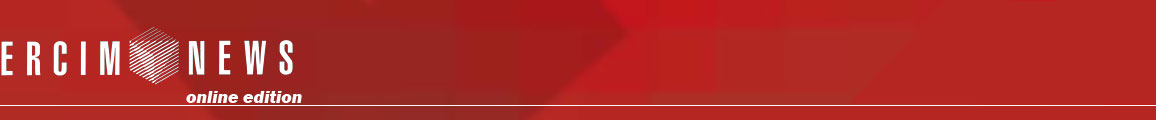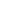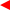contents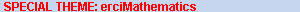ERCIM News No.50, July 2002# Theorema: A System for Computer-Supported Formal Mathematics

by Bruno Buchberger

The software system Theorema provides a uniform logic and software technologic frame for proving, solving, and simplifying formulae in all areas of mathematics. Theorema is developed at the Research Institute for Symbolic Computation (RISC), Austria.

Theorema was designed by this author, and is under development since 1996. It aims at supporting all phases and aspects of mathematical exploration: defining concepts, stating theorems, formulating algorithms, proving theorems, solving constraints, simplifying formulae, executing algorithms, composing and manipulating large mathematical knowledge bases. The logic and language frame of Theorema is a version of higher order predicate logic. Theorema is programmed in Mathematica so that it can be used on all platforms on which Mathematica is available.

Theorema provides a growing library of provers, solvers, and simplifiers for general predicate logic and various special mathematical theories (set theory, elementary analysis, various inductive domains, combinatorial identities, boolean combinations of complex equalities, coordinate geometry, and others). For most of the provers, Theorema also provides human-readable output and emphasis is laid on generating proofs whose structure and presentation resembles the style mathematicians typically follow when generating and presenting proofs.

For proving theorems in certain areas of mathematics, Theorema also applies sophisticated computer algebra methods. For example, for geometric theorem proving, the Gröbner bases method, the characteristic sets method, the area method, and Collins’ cylindric algebraic decomposition method are used. The application of such methods has two aspects: the methods are applied for proving theorems but they also need to be proved correct! The proof of the correctness of such methods is on a layer (object layer) which is different from the layer of applying the method (meta-layer) for proving. For example, exploring the theory of Gröbner bases needs set theory proving, inductive proving, and other proof techniques, whereas the application of the Gröbner bases method for geometric theorem proving proceeds in the frame of the boolean and equational theory over fields. The transition from the object layer to the meta-layer seems to be an important technique that makes human theory exploration and proving efficient and feasible. For this reason, in Theorema, emphasis is put on providing a frame for doing formal mathematics in sequences of formal layers.

Another application of this principle in Theorema makes it possible to prove the correctness of an algorithm in a first phase and then, in a second phase, to execute the algorithm without leaving the logic and language frame of Theorema. In fact, in Theorema, algorithms are just special logic formulae.

Theorema also has an elaborate functors construct by which towers of mathematical domains can be generated. Following the principle of switching between object and meta-layers, functors in Theorema are not only used for generic programming and computing in towers of domains but also for constructing corresponding towers of provers.

Theorema also provides various tools for organizing and structuring the proofs automatically generated by the system so that studying such proofs should be easier than studying human generated proofs. Two of these techniques are the ‘focus windows’ technique by which, in a given proof step, all and only the relevant formulae are displayed and the ‘logicographic symbols’ technique by which pictures can be introduced for logic predicates and functions.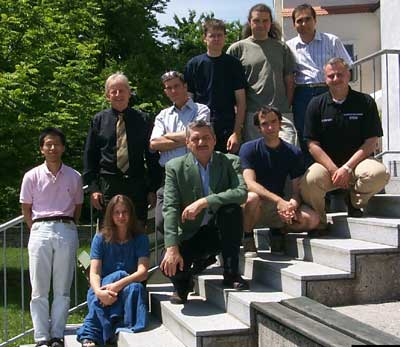The Theorema Group 2002: Standing: Koji Nakagawa, Bruno Buchberger, Wolfgang Windsteiger, Christian Vogt, Adi Craciun, Temur Kutsia. Sitting: Florina Piroi, Tudor Jebelean, Gabor Kusper, Nikolaj Popov.

The objective of Theorema is necessarily open-ended. We try to incorporate more and more proving, solving, and simplifying algorithms for various parts of mathematics and to build up a network of interactions between these algorithms. Also, equally important, hierarchies of mathematical knowledge in towers of domains is being built up. Currently we are working on two benchmark application problems: first, a formal development of the theory of Gröbner bases, which — since its introduction by B. Buchberger in the late sixties — has traditionally been a research topic at RISC, and second, a formal development of the theory of Hilbert spaces, which is the frame for joint research with numerical analysts in the project ‘Scientific Computing’ at the Johannes Kepler Uni-versity of Linz, to which RISC belongs. Also, within this project, there are particularly strong ties to the work of Peter Paule’s group on combinatorial identities. The Theorema Group is also a founding member of the European Calculemus Network in which all European groups working in the interaction between computer algebra and automated theorema proving cooperate.